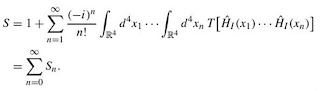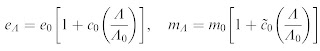## Sunday, August 07, 2011

### Renormalization in quantum field theory

So what exactly is renormalization in quantum field theory? Well, quantum field theory makes experimentally verified predictions about collisions between particles. In particular, it makes predictions about the probability of going from a particular incoming state to a particular outgoing state, and these are called transition probabilities:An incoming particle is represented by a quantum state Ψi, the interaction process is represented by a scattering operator S, and the potential outgoing state is represented by the quantum state Ψf.

In many physically relevant situations, the incoming state has a specific energy Ei and momentum ki, and each possible outgoing state also has a specific energy Ef and momentum kf. An outgoing state with a specific momentum kf, also has a specific direction Ω associated with it.

These transition probabilities can be used to construct cross-section data. The cross-section for a reaction is effectively an expression of its probability. In practice, cross-sections provide an economical way of bundling the transition probabilities between entire classes of quantum states. For example, the differential cross-section σ(E,Ω) is proportional to the probability of a transition from any incoming state Ψi of energy E to any outgoing state Ψf in which the momentum vector kf points in the direction of Ω. Integrating a differential cross-section over all possible directions then gives a total scattering cross-section σ(E).

So, what about the scattering operator S? Well, this contains the information that specifies the nature of the interaction. The nature of the interaction is specified using objects from classical physics, either the interaction Hamiltonian or the interaction Lagrangian. The interaction Lagrangian will contain values for the masses and charges (aka coupling constants) of the interacting fields. The scattering operator can be expressed in terms of the interaction Hamiltonian density operator HI(x), which in turn, can be obtained from the interaction Lagrangian density. To be specific, the scattering operator can be expressed as the following Dyson perturbation series:T[HI(x1),...,HI(xn)] simply denotes a time-ordered permutation of the interaction Hamiltonian density operators.

Inserting the expression for the scattering operator into the expression for a transition probability, yields an infinite series, and the trouble is that every term in this series transpires to be a divergent integral. Renormalization involves taking only the first few terms in such a series, and then manipulating the integrals in those terms to obtain finite results.

The most sophisticated account of renormalization goes as follows. The troublesome integrals tend to be integrals over an infinite energy range, and the integrals go to infinity as the energy goes to positive infinity. So begin by introducing a cut-off Λ0 at a large, but finite energy. Correlate this cut-off with a particular conventional interaction Lagrangian, with conventional values for the masses and coupling constants. Now stipulate that the masses and coupling constants are functions of the cut-off energy Λ. Thus, as the upper limit of the integral is now permitted to go to infinity, Λ → ∞, the masses and coupling constants becoming running masses and coupling constants, m(Λ) and g(Λ), and the Lagrangian also acquires evolving counter-terms which incorporate those running masses and coupling constants. The functional forms of m(Λ) and g(Λ) are chosen to ensure that the integrals are now finite as the limit Λ → ∞ is taken.

Thus, for example, in the case of quantum electrodynamics, the Lagrangian is modified as follows:The charge and mass have the following running values (c0 and its tilde-counterpart being proportional to ln (Λ/Λ0):This is called the Renormalization Group (RG) approach. It basically amounts to saying that there is a flow in the space of Lagrangians under energy-scale transformations. Changing the cut-off in divergent integrals is then seen to be equivalent to adding/subtracting extra terms in the Lagrangian, which in turn is equivalent to changing the values of the masses and coupling constants. There are, of course, numerous qualifications, exceptions and counter-examples, but that is the basic idea.

At a classical level in mathematical physics, the equations of a theory can be economically specified by a Lagrangian, hence it is typical in physics to identify a theory with its Lagrangian. Thus, a flow in the space of Lagrangians is also a flow in the space of theories; the RG approach is saying that different theories are appropriate at different energy scales.

I'm indebted here to the material in the following couple of papers, which also constitute excellent further reading for the enquiring mind:

Hartmann, S. (2001). Effective field theories, reductionism and scientific explanation, Studies in the History and Philosophy of Modern Physics, 32, pp267-304.

Huggett, N. and Weingard, R. (1995). The Renormalisation Group and Effective Field Theories, Synthese, Vol. 102, No. 1, pp. 171-194.